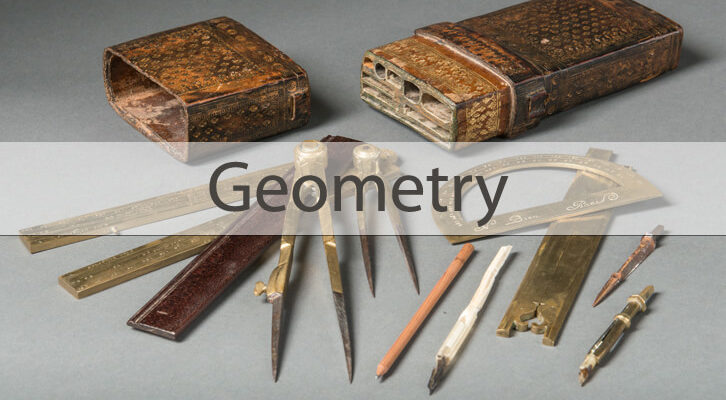# Earn your Discount: Tricky triangles – Solutions and Responses

The Earn your discount question on triangles was even more intriguing than the one on prime numbers. Each triangle has 3 angles and 3 sides. If there were two triangles that have their corresponding three angles and three sides equal, then these two are congruent. This much we know. Now, what is the maximum number among these 6 metrics – 3 angles and 3 sides – that can be equal between two triangles and the triangles still be non-congruent. To be more explicit, we can have two triangles measuring 5, 6, 7 and 5, 6 and 8. These two have one side equal and are still not congruent. We can have 2 triangles with angles 80-40-60 but with different sizes – these two are also non-congruent. Provide two triangles that have the maximum number of metrics identical but are still not-congruent

Here is an interesting cross-section of the responses we got. Many thanks to Pratyush, Vamsigupta, Vishnu Sai, Sashank, Akarsh, Joel, Akanksa and a few others for contributing solutions.

Solution 1: This is my favourite explanation, courtesy Pratyush.

A quick glance at the puzzle would make one recollect that 4 equal corresponding metrics of two triangles (out of 3 angles and 3 sides) make them congruent. Which makes one wonder why would this puzzle be worth an extra 15% discount! Obviously, the answer is not 3. And the trick lies in the fact that we are not looking for corresponding metrics. (My favourite line overall – Rajesh Balasubramanian)

Allow me to explain.

We are looking for two triangles that have the maximum number of metrics identical but are still not congruent.

Let’s consider two triangles which are similar to each other. Therefore, their corresponding angles are equal.

Triangles ABC and DEF be the two similar triangles. Angle A = Angle D, Angle B = Angle E & Angle C = Angle F.

Let the ratio of sides AB, BC and AC be x:y:z and let x>y>z. Now, the ratio of DE, EF and DF would also be x:y:z

Also, let AB = k * x

BC = k * y

AC = k * z

Similarly, DE = m * x

EF = m * y

DF = m * z

Here, k and m are constants.

Let DEF be the smaller triangle, i.e. let m < k

If we increase m such that m=k, then all 3 sides of triangle ABC would be equal to DEF, which would make it congruent. Definitely not what we want.

But is there a way to increase m such that m < k still holds true but two sides of triangle ABC become equal to two sides of triangle DEF? Yes, there is.

Let the ratio of x/y = y/z i.e. y2 = x * z.

Longest Side * Shortest Side = (Third side)2

Now, let’s increase DE such that it equals BC. That is, m*x = k * y

The other two sides of triangle DEF would also increase in the same proportion.

EF increases to ((m * y) * (k * y))/(m * x) = (k * y2)/x

From above, we know that y2 = x * z

So, EF increases to (k * x * z)/x = k * z = AC

The 3 angles are equal and now we have proven that 2 of the sides are also equal. Hence, a maximum of 5 of the metrics can be equal in a set of two triangles and the triangle may still not be congruent.

Examples of this can be a triangle with 9, 12 , 16 as its sides and another triangle with 6.75, 9, 12 as its sides. These two triangles are similar so all 3 angles are equal. That makes a total of 5 equal metrics. Do note that the sides that are equal are not corresponding sides.

This is one of my all-time favourite questions. This is never going to be tested in CAT. But if you try this one for 10 minutes, chances are you will then get absolutely convinced that this Rajesh bugger is crazy and that conventional wisdom that says “Two similar triangles with one side equal automatically become congruent” is true. Then, if you spend another 10 minutes and say ‘dammit, there is perhaps something in this’, you will get two key benefits – 1) an Aha moment that is worth is something that even Mastercard cannot buy and 2) A crystal clear understanding of congruent and similar triangles that is worth having solved 50 questions on that topic.